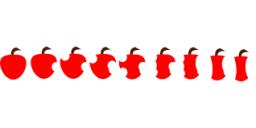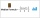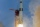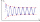# Sum of members

What is the sum of the first two members of the aritmetic progression if d = -4.3 and a3 = 7.5?

s =  27.9

### Step-by-step explanation:Did you find an error or inaccuracy? Feel free to write us. Thank you!## Related math problems and questions:

• Median or middleThe number of hours of television watched per day by a sample of 28 people is given below: 4, 1, 5, 5, 2, 5, 4, 4, 2, 3, 6, 8, 3, 5, 2, 0, 3, 5, 9, 4, 5, 2, 1, 3, 4, 7, 2, 9 What is the median value?
• Geometric sequence 4It is given geometric sequence a3 = 7 and a12 = 3. Calculate s23 (= sum of the first 23 members of the sequence).
• SelectSelect the statement that describes this expression: 5 x (3 + 4) - 4. a) 5 times the sum of 3 and 4, then subtract 4 b) 5 times 3 plus 4 minus 4 c) 5 more than the sum of 3 and 4, and then subtract 4 d) 5 times 3 plus the difference of 3 and 4 What is the
• Sequence 3Write the first 5 members of an arithmetic sequence: a4=-35, a11=-105.
• SequenceWrite the first 7 members of an arithmetic sequence: a1=-3, d=6.
• Intersect and conjuctionLet U={1,2,3,4,5,6} A={1,3,5} B={2,4,6} C={3,6} Find the following. 1. )AUB 2. )A'UB'
• Five membersWrite first 5 members geometric sequence and determine whether it is increasing or decreasing: a1 = 3 q = -2
• In theIn the arithmetic sequence a1 = 4.8, d = 0.4. How many consecutive members, starting with the first, need to be added so that the sum is greater than 170?
• AP - simpleFind the first ten members of the sequence if a11 = 132, d = 3.
• Three numbersFind three numbers so that the second number is 4 times greater than the first and the third is lower by 5 than the second number. Their sum is 67.
• Fifth memberDetermine the fifth member of the arithmetic progression, if the sum of the second and fifth members equal to 73, and difference d = 7.
• The sumThe sum of the first 10 members of the arithmetic sequence is 120. What will be the sum if the difference is reduced by 2?
• Octahedron - sumOn each wall of a regular octahedron is written one of the numbers 1, 2, 3, 4, 5, 6, 7 and 8, wherein on different sides are different numbers. For each wall John make the sum of the numbers written of three adjacent walls. Thus got eight sums, which also
• Investment 2Jack invested \$5000 in a 5-month term deposit at 4.7% p. A. . At the end of the 5-months, jack reinvested the maturity value from the first deposit into an 11-month term deposit at 7.3% p. A. What is the maturity value at the end of the second term deposi
• Special sequenceWhat if            2×9=1            3×9=2            4×9=3            5×9=4            6×9=5            7×9=6            8×9=7            9×9=8           10×9=9    then 1×9=??? answer with solutions. .. ..
• Geometric progressionIn geometric progression, a1 = 7, q = 5. Find the condition for n to sum first n members is sn≤217.
• Sequence 2Write the first 5 members of an arithmetic sequence a11=-14, d=-1